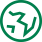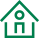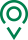• EN
• 圣康首页
• {if condition="\$categorys[\$vo]['is_menu'] == 1"}
• 关于圣康
• {if condition="\$categorys[\$vo]['is_menu'] == 1"} {if condition="\$categorys[\$vo]['is_menu'] == 1"}
• 产品展示
• {if condition="\$categorys[\$vo]['is_menu'] == 1"} {if condition="\$categorys[\$vo]['is_menu'] == 1"}
• 荣誉资质
• {if condition="\$categorys[\$vo]['is_menu'] == 1"} {if condition="\$categorys[\$vo]['is_menu'] == 1"}
• 新闻资讯
• {if condition="\$categorys[\$vo]['is_menu'] == 1"} {if condition="\$categorys[\$vo]['is_menu'] == 1"}
• 联系我们
• {if condition="\$categorys[\$vo]['is_menu'] == 1"} {if condition="\$categorys[\$vo]['is_menu'] == 1"}
• 在线留言
• {if condition="\$categorys[\$vo]['is_menu'] == 1"} {if condition="\$categorys[\$vo]['is_menu'] == 1"}
• 质量管控
• {if condition="\$categorys[\$vo]['is_menu'] == 1"} {if condition="\$categorys[\$vo]['is_menu'] == 1"}
• 源头种植
• {if condition="\$categorys[\$vo]['is_menu'] == 1"} {if condition="\$categorys[\$vo]['is_menu'] == 1"}
• 过程控制
• {if condition="\$categorys[\$vo]['is_menu'] == 1"}

新一批农业植物新品种保护名录征求意见，包括韭菜香菜

记者发现，在第十一批农业植物新品种保护名录中，共涉及51个属和种，其中不乏公众比较常见的韭菜、芫荽（别名香菜）、平菇、金针菇、满天星及金银花等。

记者从农业农村部了解到，为加大植物新品种保护力度，自1999年6月16日第一批农业植物新品种保护名录发布起，目前已正式发布十批保护名录，扩大保护范围至138个属（种），涵盖了主要粮食和经济作物。

在保护植物新品种方面，我国20余年在诸多方面采取措施。1997年颁布实施的《植物新品种保护条例》对我国植物新品种保护、激励育种创新、加快良种推广、 推动民族种业发展方面起到了重要作用；1999年4月23日，我国加入国际植物新品种保护联盟（UPOV）。截至2018年6月底，农业农村部共受理国内外品种权申请24145件，授予品种权10981件；去年年申请量达到3842件，位居国际植物新品种保护联盟（UPOV）成员第一位，我国已成为植物新品种保护大国。

我们的宗旨

•全球精选

一站式购物方案

•多仓直发

特有次日达配送服务

•源头直采

精选正宗好食材

•天天底价

新鲜到家购不停